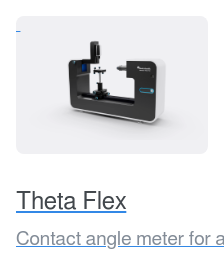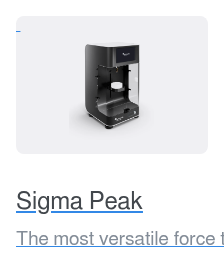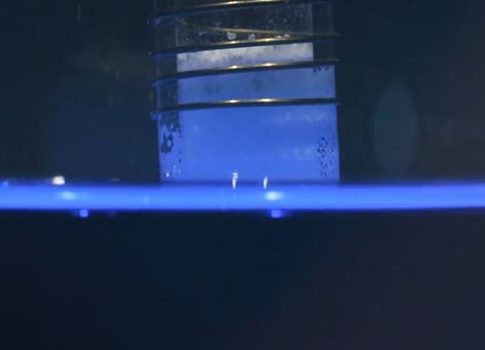# Powder Wettability

Powders have a significant role in many applications such as coatings, pharmaceutical drugs, and food products. The wettability of the powder is important when the processes and products are being developed. Powder wettability is determined through contact angle measurements.

## Basics of Powder Wettability

Powder wettability is important in applications such as coatings, dispersion, granulation processes, and other practical usages. The industrial sectors that may benefit from powder wettability studies range from pharmaceutical to mining. There is thus a need to be able to determine the contact angle between the liquid and the powder. The most commonly used contact angle measurement method is called sessile drop where a drop of liquid is placed on a solid surface. The method is straightforward for smooth, solid surfaces. With powders, there are several challenges such as surface roughness and liquid penetration into pores which makes the direct contact angle measurements difficult. For this reason, different measurement methods for the contact angle of powders have been developed and evaluated.## Contact Angle Measurement of Powders with Force Tensiometer

The wettability of loose powders and pigments is often evaluated by the sorption measurement of the liquid. A force tensiometer is utilized to detect the liquid penetration into the powder.

The power is packed on the powder holder which can be made of either glass or stainless steel. The holder is hanged on a very sensitive balance and then slightly immersed into the liquid. As the holder touches the surface of the liquid, the balance is zeroed after which the penetration of the liquid into the powder is recorded. Comparison of the speed at which the liquid is adsorbed gives some insight into the wetting properties of the powder but more common is the use of the Washburn equation to calculate the contact angle.

Washburn equation is written as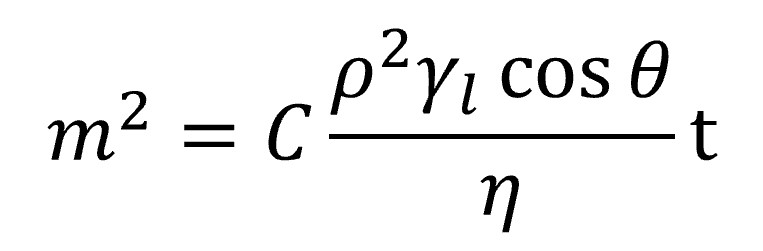, where w is the measured mass, C is material constant, ρ is the density of the liquid, γl is the surface tension of the liquid, η viscosity of the liquid, t measured time, and θ contact angle.

An experiment is performed where the mass of the adsorbed liquid is measured against time. The graph should give a straight line which slope is: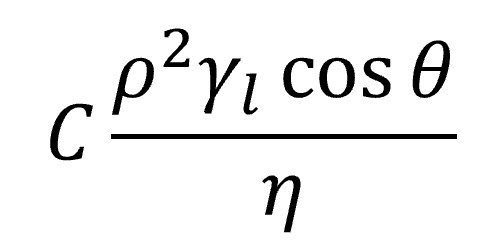If viscosity, density, and surface tension of the liquid are known then there are two unknowns left in this term, contact angle and C, the material constant for the solid. To resolve the equation, one must perform a measurement with the completely wetting liquid. If the liquid completely wets the solid, the contact angle can be assumed to be zero. N-alkanes C6 – C10 are most typically used. Liquids like methanol, ethanol, or pentane, although most probably would wet the solid completely, might be too volatile. Thus, liquids like hexane, heptane, and octane are often used. To determine the most suitable complete wetting liquid for your powder, it is recommended that you run a series of tests to find the most wettable one.

The material constant C can then be calculated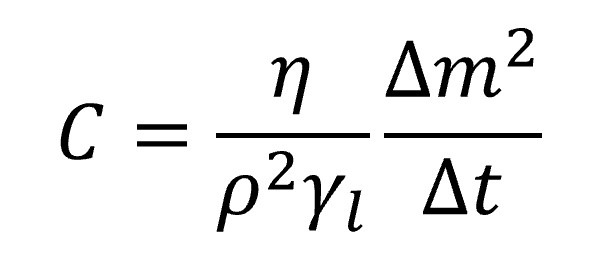It is advisable to run the test at least five times to make sure you are able to get reproducible values for the material constant C. If the values vary a lot, this means that the packing of the powder into the holder is inconsistent. To get the packing of the powder consistent is mandatory as the material constant C depends on the packing density and distribution. If the material constant varies from measurement to measurement, the contact angle value for the powder cannot be reliably determined.

Once the material contact C is determined, the same packing method needs to be used to pack the powder for the actual contact angle measurements.

Most often the contact angle measurement is done against water. Here, an important note is that if the powder is hydrophobic, i.e. the contact angle with water is higher than 90 degrees, the method described cannot be used. This is since the liquid penetration happens through capillary action which is not possible with the contact angles above 90 degrees.

Another sometimes used method for powder wettability is the Wilhelmy plate. To use the Wilhelmy plate method, the powder needs to be either compressed into a thin disc or added as a coating on a glass slide with known dimensions. After the sample preparation step, the method is pretty straightforward as the measurement is done automatically by the software. One difficulty in the measurement is the determination of the true perimeter of the sample. An accurate perimeter is needed to calculate the contact angle. Due to this and somewhat difficult sample preparation, this method is not as common as the Washburn method or the sessile drop method discussed next.

## Contact Angle Measurement of Powders with Optical Tensiometer

An optical tensiometer can be also used to study the wettability of the powder. A sessile drop method is used where a drop of liquid is placed on the powder bed. The method is relatively straightforward as there is no need to use any reference liquid, but the contact angle can be directly determined. However, the packing of the powder is something that needs to be considered. Quite often the powder is compressed into a table after which the contact angle can be measured similarly as with the standard solid sample. One of the main advantages is that there is no need to use reference liquid as the contact angle can be directly visualized. The fast penetration of water can cause problems especially if the powder is very hydrophilic. Other possibilities for sample preparation are packing the powder into a multi-well plate or sticking the powder on a glass slide with the help of an adhesive.

Surface roughness can play a significant role when porous samples are measured. It can thus be advantageous to be able to measure the surface roughness at the same location as where the contact angle is being measured. This allows for the determination of the roughness corrected contact angle.## Surface Free Energy Measurements on Powders

With the methods discussed above, it is also possible to determine the surface free energies of the powders. To calculate the surface free energy, you need to measure the contact angle of the powder with at least two liquids with the known properties. Typically, water is used as polar and di-iodomethane as a dispersive liquid.

Both optical and force tensiometers can be used to measure the surface free energy of the powder. However, one should note that the contact angles used for calculations are not the same which means that the surface free energies obtained with different methods are not comparable. With the optical method, the measured static contact angles are used for calculations. However, with the force method, there are two contact angles; advancing and receding. You then need to decide which one is more representative in your application, calculate two surface free energy values, or take the average of the two contact angles and use that for surface free energy calculations.

## Related products